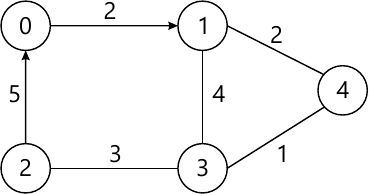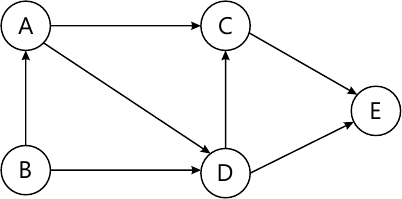MORE IN Data Structures & Algorithms
SPPU Electronics and Telecom Engineering (Semester 3)
Data Structures & Algorithms
December 2013
Total marks: --
Total time: --
INSTRUCTIONS
(1) Assume appropriate data and state your reasons
(2) Marks are given to the right of every question
(3) Draw neat diagrams wherever necessary

Solve any one question from Q1 and Q2
1 (a) Write an algorithm for searching an element in a list of integers using binary search. Discuss the time complexity of algorithm in best case and worst case.
6 M
1 (b) Explain with suitable examples, how do you pass structure variable to a Function.
6 M

2 (a) Write a function to sort the numbers in a list of integers using insertion sort. Discuss the time complexity of insertion sort algorithm in best case and worst case.
6 M
2 (b) What is subalgorithm? What are its types? Write a subalgorithm to find n!.
6 M

Solve any one question from Q3 and Q4
3 (a) Write pseudo-code to create a singly linked list of real numbers.
6 M
3 (b) What is priority queue? What are various ways of implementing priority queue? Explain any one.
6 M

4 (a) Explain following:
i) Garbage collection.
ii) Garbage compaction.
6 M
4 (b) Convert following expression into postfix format show all steps and stack contents. During every step. (a+(b*c/d)-e).
6 M

Solve any one question from Q5 and Q6
5 (a) Explain with suitable example how will you represent a binary tree using Array?
4 M
5 (b) Write psuedo-code to insert an element in a binary search tree implemented using linked representation.
5 M
5 (c) What is threaded binary tree? Create a threaded binary tree for following data. Which is BSF traversal of the tree. 10 20 30 40 50.
4 M

6 (a) The preorder and inorder traversal of a tree are given below. Draw the binary tree. Show all steps. Inorder traversal : A B C D E
Preorder traversal : A B C D E
4 M
6 (b) Create a binary search tree for following data. Show all steps. MAN, CAR, BAG, SUN, TAN.
5 M
6 (c) What is AVL tree? Explain with suitable example the RR rotation & balance factor.
4 M

Solve any one question from Q7 and Q8
7 (a) Write a functions to implement DFS traversal of graph implemented using adjacency matrix.
5 M
7 (b) Using Prim's algorithm find the minimum spanning of the graph given below.4 M
7 (c) Write topological sort for following graph.4 M

8 (a) Draw the adjacency list of the graph given in Fig. Q7). b)
4 M
8 (b) Using Kruskal's algorithm find the minimum spanning tree of the graph given in Fig. Q7). b).
4 M
8 (c) Write an algorithm to find indegree and outdegree of a vertex in a given graph.
5 M

More question papers from Data Structures & Algorithms